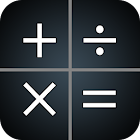Scientific Calculator free

• 검색 유형

모든 Android 애플리케이션 카테고리

모든 Android 게임 카테고리# Scientific Calculator free

by: 9.7K 9

9 사용자
평가 등급

## 스크린샷

설명

This has following features

* All Basic Mathematical Operations
* Trigonometric operations
* Hyperbolic operations
* Logarithmic operations
* Complex number operations
* Matrix Operations
* 10 variables
* HEX,DEC,OCT,BIN operations
* Fractions Support
* Degree,Minute,Second Calculations
* Solving Linear equations
* Solving Polynomial equations
* Plot Graphs
* Common unit conversions
* Predefined Scientific Constants
* Samsung Multi Window Support

You can help us by translating the app. If you like translating, drop a mail to realmaxsoft@gmail.com

Calculator might show maximum of two ads in a day. This Calculator is totally free and please report any improvements required in future releases to realmaxsoft@gmail.com.

-- FAQ --

Is this Calculator Capable of working with fractions. Yes. This can be used as a fraction calculator. Use ‘b/c’ button to enter fractions and see help for more information.

How HEX,BIN.DEC,OCT number entered ?. Change Calculator mode to Base with Mode button.

Can this be used as a Graph Calculator? Yes. see help for more information.

How to see calculation history? Use up and down arrow keys to browse calculator history.

: 9.670 리뷰

"대단함"

9